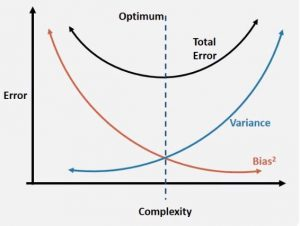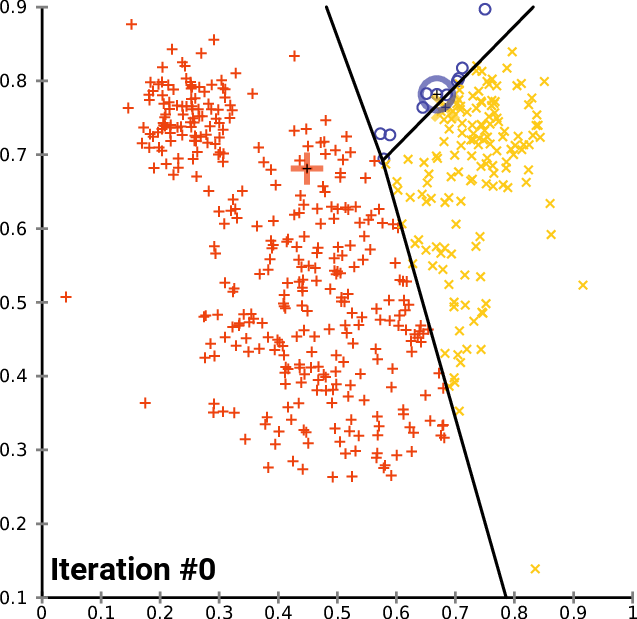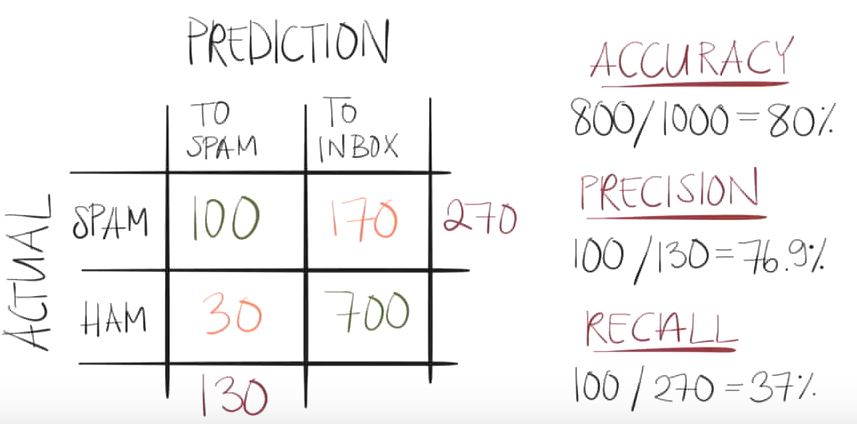# The basis

• Support vector machine (SVM) is a supervised learning method.
• It can be used for regression or classification purposes.
• SVM is useful for hyper-text categorization, classification of images, recognition of characters…
• The basic visual idea is the creation of planes (lines) to separate features.
• The position of these planes can be adjusted to maximized the margins of the separation of features.
• How we determine which plane is the best? well, it’s done using support vectors.

# Support vectors

The support vectors are the dots that determine the planes. The orange and blue dots generate lines and in the middle you can find what is called the hyper-plane.

The minimum distance between the lines created by the support vectors is called the margin.The diagram above represents the more simple support vector draw you can find, from here you can make it more complex

# Before to start

What is an error?

Observation prediction error = Target – Prediction = Bias + Variance + Noise

# The main sources of errors are

• Bias and Variability (variance).
• Underfitting or overfitting.
• Underclustering or overclustering.
• Improper validation (after the training). It could be that comes from the wrong validation set. It is important to divide completely the training and validation processes to minimize this error, and document assumptions in detail.

#Underfitting

This phenomenon happens when we have low variance and high bias.

This happens typically when we have too few features and the final model we have is too simple.

How can I prevent underfitting?

• Increase the number of features and hence the model complexity.
• If you are using a PCA, it applies a dimension reduction, so the step should be to unapply this dimension reduction.
• Perform cross-validation.

# Overfitting

This phenomenon happens when we have high variance and low bias.

This happens typically when we have too many features and the final model we have is too complex.

How can I prevent overfitting?

• Decrease the number of features and hence the complexity of the model.
• Perform a dimension reduction (PCA)
• Perform cross-validation.

# Cross validation

This is one of the typical methods to reduce the appareance of errors on a machine learning solution. It consist on testing the model in many different contexts.

You have to be careful when re-testing model on the same training/test sets, the reason? this often leads you to underfitting or overfitting errors.

The cross-validation tries to mitigate these behaviors.

The typical way to enable cross validation is to divide the data set in different sections, so you use 1 for testing, and the others for validations. For instance, you can take a stock data set from 2010 to 2017, use the data from 2012 as testing dataset and use the other divisions by year for validation of your trading model.

# Neural networks

They can be used to avoid that errors are backpropagated. The neural network helps you to minimize the error by adjusting the impact of the accumulation of data.

# The basis

• K-means clustering is an unsupervised learning method.
• The aim is to find clusters and the CentroIDs that can potentially identify the
• What is a cluster? a set of data points grouped in the same category.
• What is a CentroID? center or average of a given cluster.
• What is “k”? the number of CentroIDs

# Typical questions you will face

• The number k is not always known upfront.
• First look for the number of CentroIDs, then find the k-values (separate the 2 problems).
• Is our data “clusterable” or it is homogeneous?
• How can I determine if the dataset can be clustered?
• Can we measure its clustering tendency?

#A real situation: identification of geographical clusters

You can create a K-means algorithm, where the distance is used to know the similarities or dissimilarities. Any pointers how a properties of observations are mapped so that you can decide the groups based on the K-means or hierarchical clustering.

Since k-means tries to group based solely on euclidean distance between objects you will get back clusters of locations that are close to each other.

To find the optimal number of clusters you can try making an ‘elbow’ plot of the within group sum of square distance.

http://nbviewer.jupyter.org/github/nborwankar/LearnDataScience/blob/master/notebooks/D3.%20K-Means%20Clustering%20Analysis.ipynb.

# The basis

• It’s based on Bayes’ theorem (check the wikipedia link, and see how complex the decision trees could be).
• Assumes predictors contribute independently to the classification.
• Works well in supervised learning problems.
• Works with continuous and discrete data.
• Can work with discrete data sets.
• It is not sensitive to non-correlating “predictors”.

# Example: spam/ham classification# The basis

• Logistics or Logit regression.
• It’s a regression model where the dependent variable (DV) is categorical.
• Outcome follows a Bernoulli distribution.
• Success = 1 , failure = 0.
• Natural logarithm of odds ratio ln (p/1-p)… logit(p).
• Inverse log curve gives a nice “s” curve to work with.
• Equate logarithm of odds ratio with regression line equation.
• Solve for probability p

# ExampleContinue learning the basis !